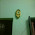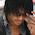# Tricks to solve Ratio and Proportion Problems

Today I'm going to discuss a very helpful trick of ratio and proportion of Quantitative Aptitude section. I'm sure this would be very helpful for you and this trick will save your more than half time, you generally take to solve the question.

## Ratio and Proportion

• Some facts of Ratio and proportion
• Ratio is written as 2:3, where 2 and 3 are known as terms.
• First term i.e. 2 is known as Antecedent.
• Second term i.e. 3 is known as Consequent.
• 2^2: 3^2 is known as duplicate ratio of 2:3.
• 2^3:3^3 is known as triplicate ratio of 2:3.
• sqrt2: sqrt3 is sub-duplicate ratio of 2:3.
• a^(1/3) : b^(1/3) is sub-triplicate ratio of 2:3.

## Ratio and Proportion Trick

Let me tell you one example which can be solved in 30 sec if you use this trick

Example: If A:B = 3: 4, B: C = 2: 3 and C:D = 5:7, then find A:B:C:D.
Solution: General method of solving this question is very lengthy, So let me tell me tell you how can we calculate it easily.

See how it is simple, you just need to remember the pattern and if you notice it, it is really simple .
Last and first steps are just the straight lines. So, what is left, just the middle pattern.
If I talk about only three terms i.e. A, B and C. Then the pattern will be much easier. Lets see how,

### Let us do an example of 5 terms:

Example: If A: B = 3: 4, B: C = 4: 5, C: D = 1: 2 and D: E = 3: 4, Find A: B: C: D: E.

Solution: Similarly, in this case, make the same pattern as in above cases:

Take a pen and try to make it yourself first or do it step by step by looking the solution. then only you can learn this technique.

A: B: C: D: E = 9: 12: 15: 30: 40
So, this is how we solve these types of questions quickly.
I will update one more session of this chapter regarding proportions.

#### What's trending in BankExamsToday

Smart Prep Kit for Banking Exams by Ramandeep Singh - Download here

##### Join 40,000+ readers and get free notes in your email1.Thank You :)

2.Very useful dude
Really u did well

1.3.It's a pressure less trick god bless you admin keep posting new tricks

4.thnx dude

5.I will try to respond asap